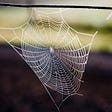# 前端刷题笔记3–11日

`nums = input()while(True):    n,m,a,b = map(int,nums.split()) //map会根据提供的函数对指定序列做映射。    dones = list(map(int,input().split()))//重新input（）获取第二行数据    minmum = min(dones)    maxmum = max(dones)    if a>b:        a,b=b,a    result = 'YES'    if minmum<a:        result = 'NO'    else:        if maxmum>b:            result='NO'        else:            if n-m==1:                if minmum>a and maxmum<b:                    result='NO'            elif n-m==0:                if minmum>a or maxmum<b:                    result='NO'    print(result)    try:        nums=input()    except:        break //如果有try就先运行try 里面的代码，如果try 中的代码出现异常，运行except 里的代码`
`nums = input()while(True):    n,x = map(int,nums.split())    score = list(map(int,input().split()))    score.sort(reverse=True)//reverse默认是false    while score[x-1]==0 and x>=1:        x=x-1    print(x)`
`nums = input()n,x = map(int,nums.split())score = list(map(int,input().split()))score.sort(reverse=True)if score[x-1]!=0 and score[x-1]==score[x]:    a=x    for i in range(a,n):        if score[a-1]==score[i]:            x=x+1    result = xelse:    while score[x-1]==0 and x>=1:        x=x-1    result = xprint(result)`
`def commonarray(N,score):    dp = [None]*N    dp=score    for i in range(1,N):        dp[i]=max(dp[i-1]+score[i],score[i])    result=max(dp)    return resultdef linkarray(N,score):    dp = [None]*N    dp=score    for i in range(1,N):        dp[i]=min(dp[i-1]+score[i],score[i])    result = sum(score)-min(dp)    return resultnums = input()while(True):    a = int(nums.split()) //nums.split()返回为字符串数组，不可以用int,所以需要取出数组中的那个数字    N = int(input().split())    score = list(map(int,input().split()))    max_common = commonarray(N,score)    max_link = linkarray(N,score)    if max_common>max_link:        result=max_common    else:        result=max_link    print(result)    try:        nums = input()    except:        break`
`nums = input()a = int(nums.split())for i in range(n):    N = int(input().split())    score = list(map(int,input().split()))    max_common = commonarray(N,score)    max_link = linkarray(N,score)    if max_common>max_link:        result=max_common    else:        result=max_link    print(result)`

--

--

--

## More from Xixibao

Love podcasts or audiobooks? Learn on the go with our new app.## 5 main points that will make anyone a flow enthusiast through cycling## Qmonos: “spiber silk” a sustainable alternative to silk fiber## “…[the album] builds over elements like a shuffle beat and lots of distortion to build its groove.”